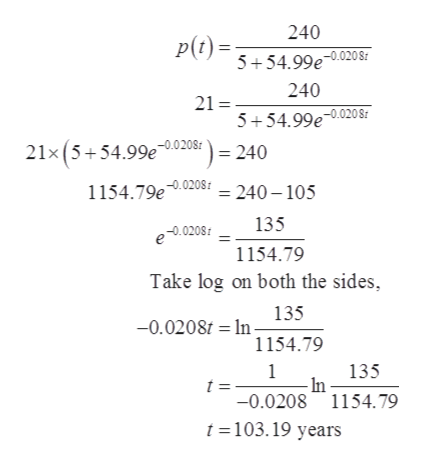# Word problemIn 1990 the populations of a country was estimated at 4 million. For any year the population is modeled by this logistic equations: p(t)= (240) ÷ (5+54.99e-.0208t) , where is the number of years since 1990. Estimate the year that the population will be 21 million.

Question
1 views

Word problem

In 1990 the populations of a country was estimated at 4 million. For any year the population is modeled by this logistic equations: p(t)= (240) ÷ (5+54.99e-.0208t) , where is the number of years since 1990. Estimate the year that the population will be 21 million.

check_circle

Step 1

To estimate the year when the population will be 21 million.

Step 2

Given:

Step 3

Substitute 21 for...help_outlineImage Transcriptionclose240 p (t)= 5 54.99e.020S 240 21= 5 54.99e-0.020 8r 0.020 8 21x (5+54.99e = 240 1154.79e.020= 240-105 135 e-0.0208 1154.79 Take log on both the sides 135 -0.0208t In 1154.79 1 In -0.0208 1154.79 135 t =- t =103.19 years fullscreen

### Want to see the full answer?

See Solution

#### Want to see this answer and more?

Solutions are written by subject experts who are available 24/7. Questions are typically answered within 1 hour.*

See Solution
*Response times may vary by subject and question.
Tagged in

### Functions